# Statistics

Statistics (from German: Statistik, orig. "description of a state, a country") is the discipline that concerns the collection, organization, analysis, interpretation, and presentation of data. In applying statistics to a scientific, industrial, or social problem, it is conventional to begin with a statistical population or a statistical model to be studied. Populations can be diverse groups of people or objects such as "all people living in a country" or "every atom composing a crystal". Statistics deals with every aspect of data, including the planning of data collection in terms of the design of surveys and experiments. When census data cannot be collected, statisticians collect data by developing specific experiment designs and survey samples. Representative sampling assures that inferences and conclusions can reasonably extend from the sample to the population as a whole. An experimental study involves taking measurements of the system under study, manipulating the system, and then taking additional measurements using the same procedure to determine if the manipulation has modified the values of the measurements. In contrast, an observational study does not involve experimental manipulation. Two main statistical methods are used in data analysis: descriptive statistics, which summarize data from a sample using indexes such as the mean or standard deviation, and inferential statistics, which draw conclusions from data that are subject to random variation (e.g., observational errors, sampling variation). Descriptive statistics are most often concerned with two sets of properties of a distribution (sample or population): central tendency (or location) seeks to characterize the distribution's central or typical value, while dispersion (or variability) characterizes the extent to which members of the distribution depart from its center and each other. Inferences on mathematical statistics are made under the framework of probability theory, which deals with the analysis of random phenomena. A standard statistical procedure involves the collection of data leading to a test of the relationship between two statistical data sets, or a data set and synthetic data drawn from an idealized model. A hypothesis is proposed for the statistical relationship between the two data sets, and this is compared as an alternative to an idealized null hypothesis of no relationship between two data sets. Rejecting or disproving the null hypothesis is done using statistical tests that quantify the sense in which the null can be proven false, given the data that are used in the test. Working from a null hypothesis, two basic forms of error are recognized: Type I errors (null hypothesis is falsely rejected giving a "false positive") and Type II errors (null hypothesis fails to be rejected and an actual relationship between populations is missed giving a "false negative"). Multiple problems have come to be associated with this framework, ranging from obtaining a sufficient sample size to specifying an adequate null hypothesis. Measurement processes that generate statistical data are also subject to error. Many of these errors are classified as random (noise) or systematic (bias), but other types of errors (e.g., blunder, such as when an analyst reports incorrect units) can also occur. The presence of missing data or censoring may result in biased estimates and specific techniques have been developed to address these problems. (Wikipedia).Statistics Lecture 3.3: Finding the Standard Deviation of a Data Set

https://www.patreon.com/ProfessorLeonard Statistics Lecture 3.3: Finding the Standard Deviation of a Data Set

From playlist Statistics (Full Length Videos)Statistics Lecture 5.2: A Study of Probability Distributions, Mean, and Standard Deviation

https://www.patreon.com/ProfessorLeonard Statistics Lecture 5.2: A Study of Probability Distributions, Mean, and Standard Deviation

From playlist Statistics (Full Length Videos)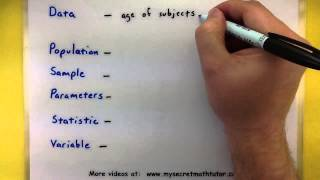Statistics - The vocabulary of statistics

This video will give show you a few terms that are used in statistics such as data, population, sample, parameter, statistic, and variable. Remember that it matters if you are talking about the whole group, or a portion of that group. For more videos please visit http://www.mysecretmatht

From playlist Statistics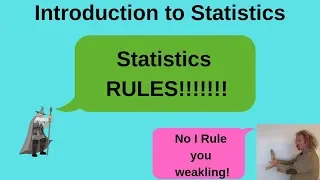Introduction to Statistics

Please Subscribe here, thank you!!! https://goo.gl/JQ8Nys Introduction to Statistics - Quantitative Data versus Qualitative Data

From playlist StatisticsWhat is Statistics?

This branch of math can help you to organize and interpret information. It’s used in a variety of fields, and it has many applications in daily life. To learn more basic concepts in #statistics, check out the free tutorial on our website: https://edu.gcfglobal.org/en/statistics-basic-conce

From playlist Basic Statistics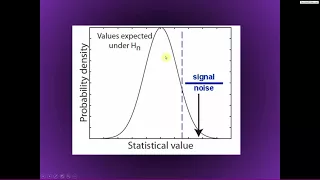Introduction to statistics

This lecturelet will introduce you to the series on statistical analyses of time-frequency data. For more online courses about programming, data analysis, linear algebra, and statistics, see http://sincxpress.com/

From playlist OLD ANTS #8) Statistics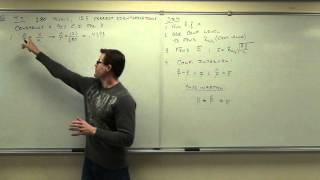Statistics Lecture 7.2: Finding Confidence Intervals for the Population Proportion

https://www.patreon.com/ProfessorLeonard Statistics Lecture 7.2: Finding Confidence Intervals for the Population Proportion

From playlist Statistics (Full Length Videos)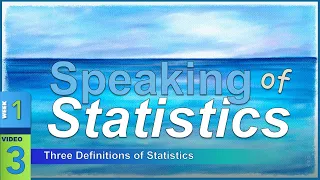Speaking of Statistics – DEFINING the Field of Statistics (1-3)

The term “statistics” may refer to the practice, procedure, or products of statistics. The Practice of Statistics means the field of statistics: “The art and science of making sense of data”. The Procedures of Statistics reefer to mathematical techniques to organize, summarize, and interprThe Vocabulary of Statistics – Terminology for Beginning Statistics (1-1)

Welcome to the study of statistics! We will begin with an introduction to some new terminology that we will be using in our adventure. I will introduce you to four goals of statistics (to collect, organize, analyze, and interpret data), three meanings of the word “statistics” (practice, prWhat Is Statistics: Crash Course Statistics #1

Welcome to Crash Course Statistics! In this series we're going to take a look at the important role statistics play in our everyday lives, because statistics are everywhere! Statistics help us better understand the world and make decisions from what you'll wear tomorrow to government polic

From playlist Back to School - Expanded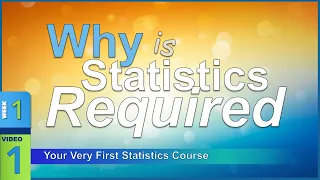Why is Statistics REQUIRED? Your Very First Statistics Course (1-1)

A variable is any characteristic that can vary. An organized collection of numbers can be a variable. Qualitative variables indicate an attribute or belongingness to a category. Dichotomous variables are discrete variables that can have two and only two values. Quantitative variables indic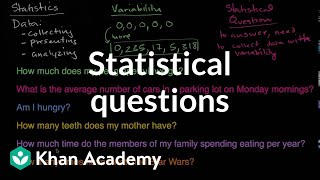Statistical questions | Data and statistics | 6th grade | Khan Academy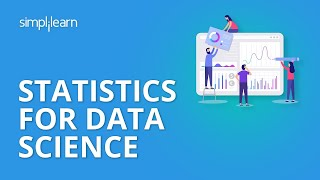Statistics For Data Science | Data Science Tutorial | Simplilearn

🔥 Advanced Certificate Program In Data Science: https://www.simplilearn.com/pgp-data-science-certification-bootcamp-program?utm_campaign=StatisticsForDataScience-Lv0xcdeXaGU&utm_medium=DescriptionFirstFold&utm_source=youtube 🔥 Data Science Bootcamp (US Only): https://www.simplilearn.com/da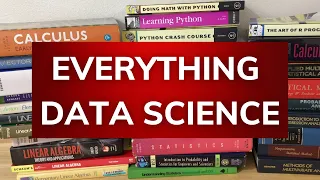Everything Data Science

In this video I will give you the resources you need to learn data science from zero knowledge. We will discuss several programming books and math books that are perfect for beginners who want to acquire the skills to become a data scientist. In particular we will look at books on R, Pytho

From playlist Book ReviewsMountainWest RubyConf 2015 - Learning Statistics Will Save Your Life

by John Paul Ashenfelter Many of the greatest achievements in the history of computers are based on lies, or rather, the strategic sets of lies we generallly call “abstraction”. Operating systems lie to programs about hardware, multitasking systems lie to users about parallelism, Ruby lies

From playlist MWRC 2015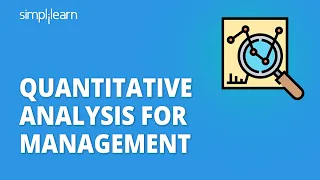Quantitative Analysis For Management | Quantitative Analysis Explained For Beginners | Simplilearn

This video on Quantitative Analysis for Management will acquaint you with all the essential details that you should know about quantitative business analysis. In this Quantitative Analysis Explained For Beginners tutorial, you will understand what quantitative analysis is. You'll also lear

From playlist Ful Stack Web Development 🔥[2023 Updated]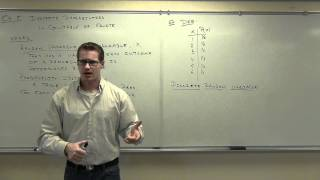Statistics Lecture 5.2 Part 1

Statistics Lecture 5.2 Part 1: Probability Distributions, Mean, and Standard Deviation

From playlist Statistics Playlist 1